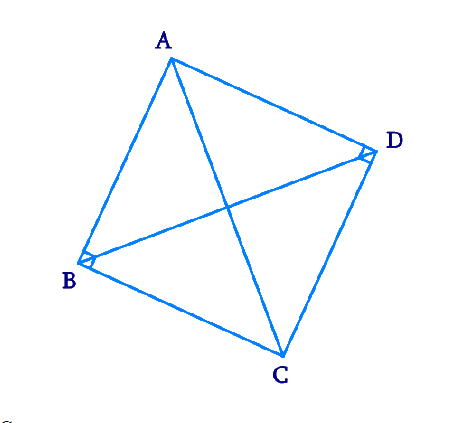# Ex.10.5 Q11 Circles Solution - NCERT Maths Class 9

Go back to  'Ex.10.5'

## Question

$${ABC}$$ and $${ADC}$$ are two right triangles with common hypotenuse $${AC.}$$ Prove that $$\angle{CAD}= \angle {CBD}.$$

Video Solution
Circles
Ex 10.5 | Question 11

## Text Solution

What is given ?

$${ABC}$$ and $${ADC}$$ are two right triangles with common hypotenuse $${AC.}$$

What is unknown?

Proof of $$\angle{CAD}= \angle {CBD}$$

Reasoning:

Sum of all angles in a triangle is $$180^\circ$$

If the sum of pair of opposite angles in a quadrilateral is $$180$$ then it is cyclic quarilateral

Angles in the same segment of a circle are equal.

Steps:Consider $$\Delta {ABC,}$$

\begin{align}&\angle {ABC}+ \angle {BCA}+\angle {CAB} =180^{\circ} \\&\text { (Angle sum property of a triangle) }\end{align}

\begin{align} 90^{\circ}+\angle {BCA}+\angle {CAB}&=180^{\circ} \\ \angle {BCA}+\angle {CAB}&=90^{\circ} \ldots(1)\end{align}

Consider $$\Delta {ADC,}$$

\begin{align}&\angle {CDA}+ \angle {ACD}+\angle {DAC} =180^{\circ} \\&\text { (Angle sum property of a triangle) }\end{align}

\begin {align} 90^{\circ}+\angle {ACD}+\angle {DAC}&=180^{\circ}\\\angle {ACD}+\angle {DAC}&=90^{\circ} \ldots(2) \end {align}

Adding Equations ($$1$$) and ($$2$$), we obtain

\begin {align} \begin{Bmatrix} \angle {BCA}+\angle {CAB}\\+\angle {ACD}+\angle {DAC} \end{Bmatrix}&=180^{\circ} \\ \begin{Bmatrix} (\angle {BCA}+\angle {ACD})+ \\ (\angle {CAB}+\angle {DAC}) \end{Bmatrix} &=180^{\circ} \end {align}

\begin {align} \angle {BCD}+\angle {DAB}&=180^{\circ} \ldots(3) \end {align}

However, it is given that

\begin {align}\angle {B}+\angle {D} &=90^{\circ}+90^{\circ} \\ &=180^{\circ} \ldots(4) \end {align}

From Equations ($$3$$) and ($$4$$), it can be observed that the sum of the measures of opposite angles of quadrilateral $$\text{ABCD}$$ is $$180^{\circ}.$$ Therefore, it is a cyclic quadrilateral.

Consider chord $${CD.}$$

\begin {align}\angle {CAD} = \angle {CBD} \end {align} (Angles in the same segment)

Video Solution
Circles
Ex 10.5 | Question 11

Learn from the best math teachers and top your exams

• Live one on one classroom and doubt clearing
• Practice worksheets in and after class for conceptual clarity
• Personalized curriculum to keep up with school